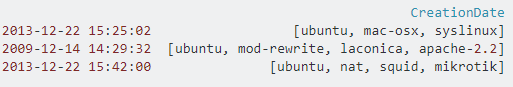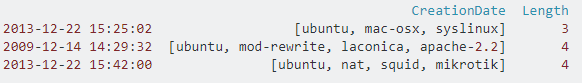2 views

I have a dataframe like this:I am calculation length of lists in the CreationDate column and making a new Length column like this:

df['Length'] = df.CreationDate.apply(lambda x: len(x))

Which gives me this:Is there a more pythonic way to do this?

by (108k points)

You can utilize the str accessor for some list operations as well. For instance:

df['CreationDate'].str.len()

returns the length of each list. Refer the following link for str.len:

https://pandas.pydata.org/pandas-docs/stable/reference/api/pandas.Series.str.len.html